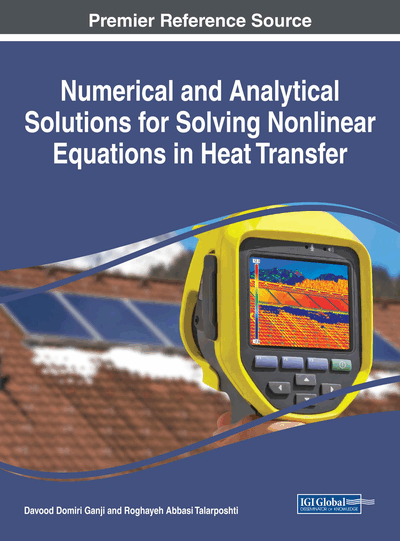# He's Variational Iteration Method

DOI: 10.4018/978-1-5225-2713-8.ch003
Available
\$37.50
No Current Special Offers

## Abstract

In this chapter, a variational iteration method (VIM) has been applied to nonlinear heat transfer equation. The concept of the variational iteration method is introduced briefly for applying this method for problem solving. The proposed iterative scheme finds the solution without any discretization, linearization, or restrictive assumptions. The results reveal that the VIM is very effective and convenient in predicting the solution of such problems.
Chapter Preview
Top

## Introduction

### Basic Idea of He's Variational Iteration Method

To illustrate the basic concepts of variational iteration method, we consider the following deferential equation:

(1) whereis a linear operator,a nonlinear operator, anda heterogeneous term. According to VIM, we can construct a correction functional as follows:(2) whereis a general Lagrangian multiplier (He, 1998a, 1999b), which can be identified optimally via the variational theory (He, 1999b), the subscriptindicates the nth order approximation,which is considered as a restricted variation, i.e..

Top

## Background

Most of engineering problems, especially some heat transfer equations are nonlinear, and in most cases it is difficult to solve them, especially analytically. Perturbation method is one of the well-known methods to solve nonlinear problems, it is based on the existence of small/large parameters, the so-called perturbation quantity (Cole, 1968; Nayfeh, 2000). Many nonlinear problems do not contain such kind of perturbation quantity, and we can use non perturbation methods, such as the artificial small parameter method (Lyapunov, 1992), the-expansion method (Karmishin, Zhukov & Kolosov, 1990), the Adomian’s decomposition method (Adomian, 1994), the homotopy perturbation method (HPM) (He, 2005, 2007; Ganji & Rajabi, 2006; He, 2006, 2000), and the variational iteration method (VIM) (He, 2000, 1998a; Ganji & Sadighi, 2006; Ganji, Jannatabadi & Mohseni, 2006).

## Complete Chapter List

Search this Book:
Reset